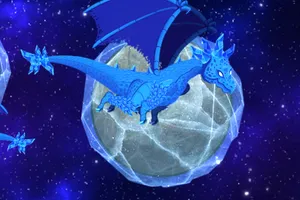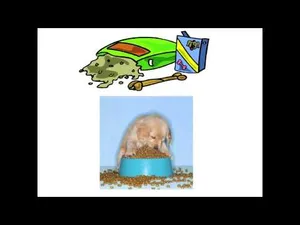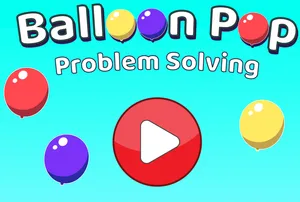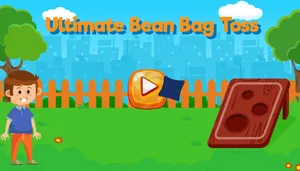# Pet City: Unknown Numbers

3rd grade | 3.OA.A.4 | 7 minutes

In this math game, 3rd graders practice filling in the blank to make division equations true.

Subject

Unlock this activity and thousands more in eSpark’s playfully personalized learning environment.

Or

Related ActivitiesTry Now

Dragon Doom

Unknown Number Equations, 3.OA.A.4Try Now

Finding the Missing Number in Equations

Unknown Number Equations, 3.OA.A.4Try Now

Balloon Pop Problem Solving

Multiply, Divide: Word Problems, 3.OA.A.3Try Now

Ultimate Bean Bag Toss

Multiply, Divide: Word Problems, 3.OA.A.3

Company